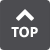##N No-Dwell Motion, Rise- Return-Rise Motion The reciprocating motion repeated from starting point to stopping point without any interruption while the stroke continues to accelerate at a given rate. Used to reduce the Am. Non-Dimensional Acceleration Non-dimensional velocity differentiated by non-dimensional time. The maximum non-dimensional acceleration is expressed as Am. For high-speed driving applications, select the curve with the smallest Am. Non-Dimensional Displacement The displacement of a motion from start to stop represented between 0 to 1. S = s/yh NOTE: S is the non-dimensional displacement, s is the real displacement (mm or rad), and yh is the maximum displacement (mm or rad). Non-Dimensional Jerk Non-dimensional acceleration differentiated by non-dimensional time. Used to indicate the rate of change in acceleration. The maximum non-dimensional jerk is expressed as Jm. Non-Dimensional Time The time of a motion from start to stop represented between 0 to 1. T = t/th NOTE: T is the non-dimensional time, t is the real time (s), and th is the time (s) up to the maximum displacement. Non-Dimensional Velocity Non-dimensional displacement differentiated by non-dimensional time. The maximum non-dimensional velocity is expressed as Vm. When driving heavy loads, select the curve with the smallest Vm. Normal Direction The vertical and perpendicular directions of a line at any given point on a plane or curve. Number of Stops The number of stops the output shaft makes during intermittent (indexing) rotation. The output shaft rotates 360°/s between stops where s equals the number of stops.